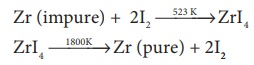Home | | Chemistry 12th Std | Metallurgy: Choose the correct answer

# Metallurgy: Choose the correct answer

1. Bauxite has the composition

a) Al2 O3

b) Al2 O3 .nH2O

c) Fe2 O3 .2H2O

d) None of these

2. Roasting of sulphide ore gives the gas (A).(A) is a colourless gas. Aqueous solution of (A) is acidic. The gas (A) is

a) CO2

b) SO3

c) SO2

d) H2S

3. Which one of the following reaction represents calcinations?

a) 2Zn + O2 → 2ZnO

b) 2ZnS + 3O2 →2ZnO + 2SO2

c) MgCO3 →MgO + CO2

d) Both (a) and (c)

4. The metal oxide which cannot be reduced to metal by carbon is

a) PbO

b) Al2O3

c) ZnO

d) FeO

5. Which of the metal is extracted by Hall-Heroult process?

a) Al

b) Ni

c) Cu

d) Zn

6. Which of the following statements, about the advantage of roasting of sulphide ore before reduction is not true?

a) ΔGf0 of sulphide is greater than those for CS2 and H2S .

b) ΔG f0 is negative for roasting of sulphide ore to oxide

c) Roasting of the sulphide to its oxide is thermodynamically feasible.

d) Carbon and hydrogen are suitable reducing agents for metal sulphides.

7. Match items in column - I with the items of column – II and assign the correct code.a) A-i , B-ii , C-iii , D-iv

b) A-iii , B-iv , C-v , D-i

c) A-iv , B-ii , C-iii , D-i

d) A-ii , B-iii , C-i , D-v

8. Wolframite ore is separated from tinstone by the process of

a) Smelting

b) Calcination

c) Roasting

d) Electromagnetic separation

9. Which one of the following is not feasible

a) Zn(s) + Cu2+ (aq) → Cu(s) + Zn2+ (aq)

b) Cu(s) + Zn2+ (aq) → Zn(s) + Cu2+ (aq)

c) Cu(s) + 2Ag+ (aq) → 2Ag(s) + Cu2+ (aq)

d) Fe(s) + Cu2+ (aq) → Cu(s) + Fe2+ (aq)

10. Electrochemical process is used to extract

a) Iron

c) Sodium

d) silver

11. Flux is a substance which is used to convert

a) Mineral into silicate

b) Infusible impurities to soluble impurities

c) Soluble impurities to infusible impurities

d) All of these

12. Which one of the following ores is best concentrated by froth – floatation method?

a) Magnetite

b) Haematite

c) Galena

d) Cassiterite

13. In the extraction of aluminium from alumina by electrolysis, cryolite is added to

a) Lower the melting point of alumina

b) Remove impurities from alumina

c) Decrease the electrical conductivity

d) Increase the rate of reduction

14. Zinc is obtained from ZnO by

a) Carbon reduction

b) Reduction using silver

c) Electrochemical process

d) Acid leaching

15. Cupellation is a process used for the refining of

a) Silver

c) Copper

d) iron

16. Extraction of gold and silver involves leaching with cyanide ion. silver is later recovered by

a) Distillation

b) Zone refining

c) Displacement with zinc

d) liquation

17. Considering Ellingham diagram, which of the following metals can be used to reduce alumina?

a) Fe

b) Cu

c) Mg

d) Zn

18. The following set of reactions are used in refining ZirconiumThis method is known as

a) Liquation

b) van Arkel process

c) Zone refining

d) Mond’s process

19. Which of the following is used for concentrating ore in metallurgy?

a) Leaching

b) Roasting

c) Froth floatation

d) Both (a) and (c)

20. The incorrect statement among the following is

a) Nickel is refined by Mond’s process

b) Titanium is refined by Van Arkel’s process

c) Zinc blende is concentrated by froth floatation

d) In the metallurgy of gold, the metal is leached with dilute sodium chloride solution

21. In the electrolytic refining of copper, which one of the following is used as anode?

a) Pure copper

b) Impure copper

c) Carbon rod

d) Platinum electrode

22. Which of the following plot gives Ellingham diagram

a) ΔSVsT

b) ΔG0Vs T

c) ΔG0 Vs 1/T

d) ΔG0 Vs T2

23. In the Ellingham diagram, for the formation of carbon monoxideAns: c) (ΔGº/ΔT) is negative

24. Which of the following reduction is not thermodynamically feasible?

a) Cr2O3 + 2Al → Al2O3 + 2Cr

b) Al2O3 + 2Cr → Cr2O3 + 2Al

c) 3TiO2 + 4Al → 2 Al2O3 + 3Ti

d) none of these

25. Which of the following is not true with respect to Ellingham diagram?

a) Free energy changes follow a straight line. Deviation occurs when there is a phase change.

b) The graph for the formation of CO2 is a straight line almost parallel to free energy axis.

c) Negative slope of CO shows that it becomes more stable with increase in temperature.

d) Positive slope of metal oxides shows that their stabilities decrease with increase in temperature.

Tags : Chemistry , 12th Chemistry : UNIT 1 : Metallurgy
Study Material, Lecturing Notes, Assignment, Reference, Wiki description explanation, brief detail
12th Chemistry : UNIT 1 : Metallurgy : Metallurgy: Choose the correct answer | Chemistry